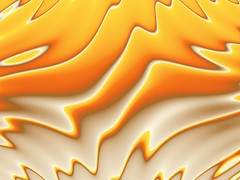# Genetic Art – Part 2

After my initial Genetic Art success I was inspired to try a formula based approach.

For Genetic Art 2 the process of creation is as follows;
1. The screen pixels are mapped to X and Y values. The default X and Y coordinates range from -5 to +5.
2. Every coordinate/pixel is fed through the current formula and all the pixel values are scaled within the range of 0-255. This allows each pixel to be assigned a color from the currently loaded color palette.

Here are a few thumbnail images and their associated formulas;arctan((y)+y-sin(y)+arctan(pi)+tan(x*y)/sin(x))y-arctan((y)-arctan(y-x)-sin(y*pi)-sqr(y)*y-sin(y*x-0.3))arctan(((y)+tan((x+y)-sin(x+pi)-sin(x*y/pi)*sin(((y*x+pi))))))

Even though I am still only mutating formulas (and not breeding 2 parent formulas) the results using a formula based approach are much more varied.

If you would like to try making your own Genetic Art, try Visions Of Chaos.

There are many more examples of what the Genetic Art 2 process can create here.

Stay tuned for Genetic Art 3. I still want to have a true tree based approach to the formulas so they can be cross-bred and mutated. So two or more parents can be combined to create similar images that have attributes of both of them. A nice example of this methodology is shown here.

Jason.